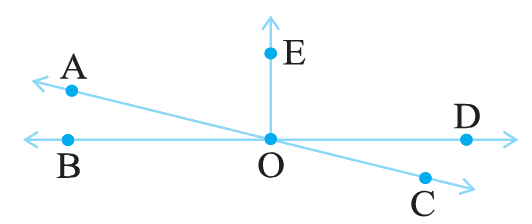# Ex.5.1 Q14 Lines and Angles - NCERT Maths Class 7

## Question

In the adjoining figure, name the following pairs of angles:

(i) Obtuse vertically opposite angles.

(ii) Adjacent complementary angles

(iii) Equal supplementary angles.

(iv) Unequal supplementary angles.

(v) Adjacent angles that do not form a linear pair.Video Solution
Lines & Angles
Ex 5.1 | Question 14

## Text Solution

Steps:

(i) Obtuse vertically opposite angles mean angles greater than $$90^\circ$$ and are equal

$$\angle AOD = \angle BOC$$

(ii) Adjacent complementary angles have common vertex and common arm, non - common arms are on either sides of common arm and their sum is $$90^\circ.$$

$$\angle EOA \,\,{\text {and}} \,\,\angle AOB$$ are adjacent complementary angles.

(iii) Equal supplementary angles have sum of angles $$180^\circ$$ and supplementary angles are equal.

$$\angle EOB\,\,{\text {and} }\,\,\angle EOD$$

(iv) Unequal supplementary angles have sum of angles $$180^\circ$$ and supplementary angles are unequal.

$$\angle EOA$$ and  $$\angle EOC.$$

(v) Adjacent angles that do not form a linear pair. (Linear pairs are adjacent angles whose sum is equal to $$180^\circ$$).

$$\angle AOB$$ and $$\angle AOE;$$ $$\angle AOE$$ and $$\angle EOD;$$ $$\angle EOD$$ and $$\angle COD$$

Learn from the best math teachers and top your exams

• Live one on one classroom and doubt clearing
• Practice worksheets in and after class for conceptual clarity
• Personalized curriculum to keep up with school